# Fluid Dynamics¶

Important: Please read the installation page for details about how to install the toolboxes. $\newcommand{\dotp}{\langle #1, #2 \rangle}$ $\newcommand{\enscond}{\lbrace #1, #2 \rbrace}$ $\newcommand{\pd}{ \frac{ \partial #1}{\partial #2} }$ $\newcommand{\umin}{\underset{#1}{\min}\;}$ $\newcommand{\umax}{\underset{#1}{\max}\;}$ $\newcommand{\umin}{\underset{#1}{\min}\;}$ $\newcommand{\uargmin}{\underset{#1}{argmin}\;}$ $\newcommand{\norm}{\|#1\|}$ $\newcommand{\abs}{\left|#1\right|}$ $\newcommand{\choice}{ \left\{ \begin{array}{l} #1 \end{array} \right. }$ $\newcommand{\pa}{\left(#1\right)}$ $\newcommand{\diag}{{diag}\left( #1 \right)}$ $\newcommand{\qandq}{\quad\text{and}\quad}$ $\newcommand{\qwhereq}{\quad\text{where}\quad}$ $\newcommand{\qifq}{ \quad \text{if} \quad }$ $\newcommand{\qarrq}{ \quad \Longrightarrow \quad }$ $\newcommand{\ZZ}{\mathbb{Z}}$ $\newcommand{\CC}{\mathbb{C}}$ $\newcommand{\RR}{\mathbb{R}}$ $\newcommand{\EE}{\mathbb{E}}$ $\newcommand{\Zz}{\mathcal{Z}}$ $\newcommand{\Ww}{\mathcal{W}}$ $\newcommand{\Vv}{\mathcal{V}}$ $\newcommand{\Nn}{\mathcal{N}}$ $\newcommand{\NN}{\mathcal{N}}$ $\newcommand{\Hh}{\mathcal{H}}$ $\newcommand{\Bb}{\mathcal{B}}$ $\newcommand{\Ee}{\mathcal{E}}$ $\newcommand{\Cc}{\mathcal{C}}$ $\newcommand{\Gg}{\mathcal{G}}$ $\newcommand{\Ss}{\mathcal{S}}$ $\newcommand{\Pp}{\mathcal{P}}$ $\newcommand{\Ff}{\mathcal{F}}$ $\newcommand{\Xx}{\mathcal{X}}$ $\newcommand{\Mm}{\mathcal{M}}$ $\newcommand{\Ii}{\mathcal{I}}$ $\newcommand{\Dd}{\mathcal{D}}$ $\newcommand{\Ll}{\mathcal{L}}$ $\newcommand{\Tt}{\mathcal{T}}$ $\newcommand{\si}{\sigma}$ $\newcommand{\al}{\alpha}$ $\newcommand{\la}{\lambda}$ $\newcommand{\ga}{\gamma}$ $\newcommand{\Ga}{\Gamma}$ $\newcommand{\La}{\Lambda}$ $\newcommand{\si}{\sigma}$ $\newcommand{\Si}{\Sigma}$ $\newcommand{\be}{\beta}$ $\newcommand{\de}{\delta}$ $\newcommand{\De}{\Delta}$ $\newcommand{\phi}{\varphi}$ $\newcommand{\th}{\theta}$ $\newcommand{\om}{\omega}$ $\newcommand{\Om}{\Omega}$

This numerical tour explores fluid dynamics for image generation.

In :
# options(warn=-1) # turns off warnings, to turn on: "options(warn=0)"

library(png)
library(pracma)
library(imager)

for (f in list.files(path="nt_toolbox/toolbox_general/", pattern="*.R")) {
source(paste("nt_toolbox/toolbox_general/", f, sep=""))
}

for (f in list.files(path="nt_toolbox/toolbox_signal/", pattern="*.R")) {
source(paste("nt_toolbox/toolbox_signal/", f, sep=""))
}

source("nt_toolbox/toolbox_wavelet_meshes/meshgrid.R")
options(repr.plot.width=5, repr.plot.height=5)


## Velocity Flow Field¶

A velocity flow is simply a 2-D vector field $V = (V_i)_{i=1}^N \in \RR^{n \times n \times 2}$ where $V_i \in \RR^2$ is one of the $N=n \times n$ vectors at a position indexed by $i$.

It can be generated as a realization of Gaussian process. The blurring creates correlations in the flow.

In :
n <- 128
V <- perform_blurring( array( rnorm(n*n*2), c(n,n,2) ), c(40), "per" )

 127 127
 127 127
 127 127
 127 127


Subsampling display operator.

In :
myplot <- function(V){ plot_vf(V[seq(1,n,6),seq(1,n,6),]) }


We can display the vector field using arrow.

In :
myplot(V)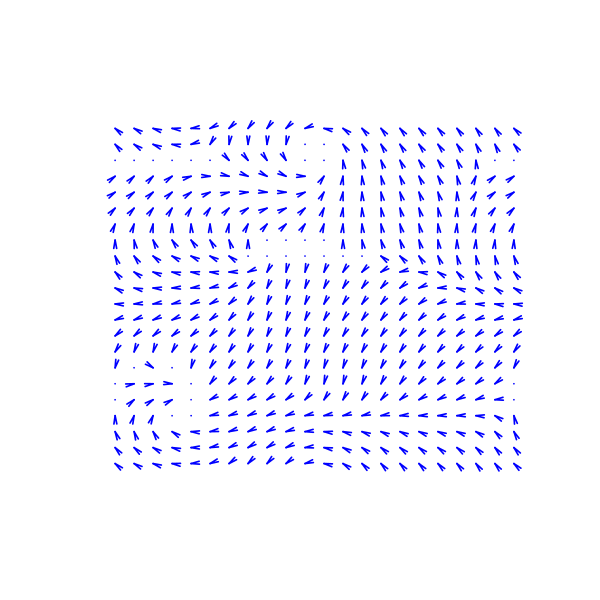We can renormalize the flow, which enhances the singularities. It defines $\tilde V$ as $\tilde V_i = V_i/\norm{V_i}$.

In :
normalize <- function(V){ V/ array(rep( pmax( array(1e-9, dim(V)[1:2]), sqrt(apply(V**2, c(1,2), sum)) ), 2 ), dim(V)) }


Display.

In :
myplot(normalize(V))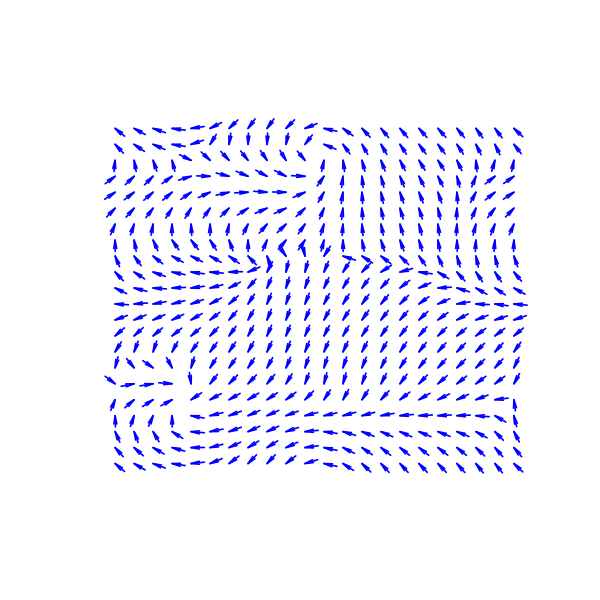## Incompressible Flows¶

An incompressible flow has a vanishing divergence. The set of vector incompressible flow defines a sub-space of $\RR^{n \times n \times 2}$ $$\Ii = \enscond{V}{ \text{div}(V)=0 } \qwhereq \text{div}(V) = \pd{V}{x_1} + \pd{V}{x_2} \in \RR^{n \times n}.$$ Here $\pd{}{x_s}$ for $s=1,2$ are finite differences approximation of the horizontal and vertical derivative operators (we suppose here periodic boundary conditions).

The orthogonal projection $U = \text{Proj}_{\Ii}(V)$ on $\Ii$ is computed by solving a Poisson equation $$U = V-\nabla A \qwhereq \Delta A = \text{div}(V).$$

This is especially simple for periodic boundary conditions since $A$ can be computed over the Fourier domain as $$\forall \om \neq 0, \quad \hat A(\om) = \frac{\hat Y(\om)}{\mu(\om)} \qwhereq Y = \text{div}(V) \qandq \mu(\om_1,\om_2) = -4 \sin(\om_1 \pi / n)^2 -4 \sin(\om_2 \pi / n)^2$$ and $\hat A(0)=0$.

Compute the kernel $\mu(\om)$.

In :
grid <- meshgrid_2d(0:(n-1), 0:(n-1))
Y <- grid$X ; X <- grid$Y
mu <- sin(X*pi/n)**2
mu <- -4*(mu + t(mu))
mu[1,1] <- 1


Computation of $A$.

In :
A <- function(V){ FFT <- fft( div(V[,,1], V[,,2], bound="per") )
return( Re( fft( FFT/mu, inverse=TRUE)/length(FFT) ) ) }


Projection on incompressible flows.

In :
ProjI <- function(V){ V - grad(A(V), bound="per") }


Display $U=\text{Proj}_{\Ii}(V)$.

In :
U <- ProjI(V)

myplot(U)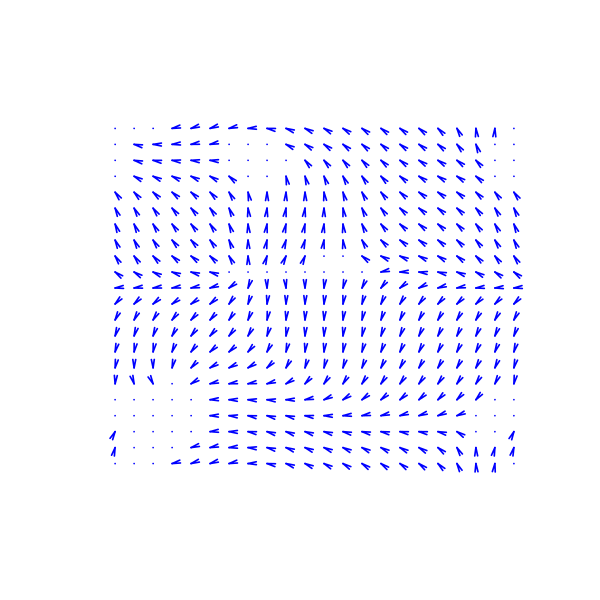Display $W=U-V$ the irrotational component of $V$.

In :
myplot(V-U)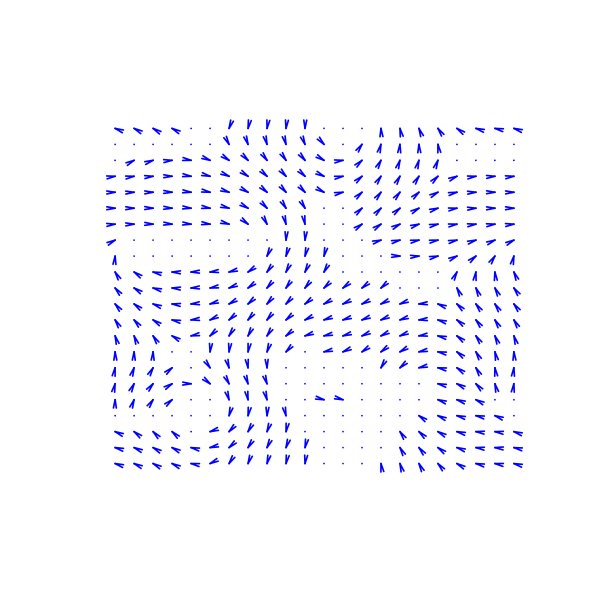Note that the decomposition $V=U+W$ is called the Hoge decomposition of the vector field.

## Image Advection Along the Flow¶

A flow defines a warping operator that transport the content of an image along the streaming of the flow.

We load an image $f$.

In :
f <- as.matrix(load_image("nt_toolbox/data/lena.png", 2*n))
f <- f[(n-round(n/2)+1):(n+round(n/2)),(n-round(n/2)+1):(n+round(n/2))]


Given some vector field $U$, the warping operator $f_1 = \Ww_U(f)$ along the flow is defined $$f_1(x) = f(x+U(x))$$ i.e. it advects the values of $f$ by the vector field $U$ to obtain the values of $f_1$.

We define $U$ as a scaled normalized incompressible flow.

In :
U <- normalize(ProjI(V))


Helper function: enforce periodicity.

In :
periodic <- function(P){ mod(P,n) }


Helper function: extend an image by 1 pixel to avoid boundary problems.

In :
extend1 <- function(f){ ext <- array(0, c(dim(f), dim(f)+1))
ext[,1:dim(f)] <- f
ext[,dim(ext)] <- f[,1]
return(ext) }

extend <- function(f){ t(extend1(t(extend1(f)))) }


Helper function: bilinear interpolation on a grid.

In :
myinterp <- function(f1, Pi){
dim_f1 <- dim(f1)
f1 <- as.cimg(t(f1))
locations <- data.frame(x=as.vector(Pi[,,2]), y=as.vector(Pi[,,1]))
return( as.matrix(as.cimg(imager::interp(f1, locations))) )
}


First we compute the initial and wraped grids.

In :
grid <- meshgrid_2d(1:n, 1:n)
Y <- grid$X ; X <- grid$Y
P <- array(0, c(dim(X),2)) ; P[,,1] <- X ; P[,,2] <- Y


Defines the warping operator $\Ww_U$.

In :
W <- function(f, U){ myinterp(extend(f), periodic(P - U)) }


Display a warped image $\Ww_{\rho U}(f)$ for some scaling $\rho$.

In :
rho <- 2
options(repr.plot.width=5, repr.plot.height=5)
imageplot(W(f, rho*U))Exercise 1

Display $\Ww_{\rho U}(f)$ for various values of $\rho$.

In :
options(repr.plot.width=7, repr.plot.height=7)

source("nt_solutions/graphics_5_fluids/exo1.R")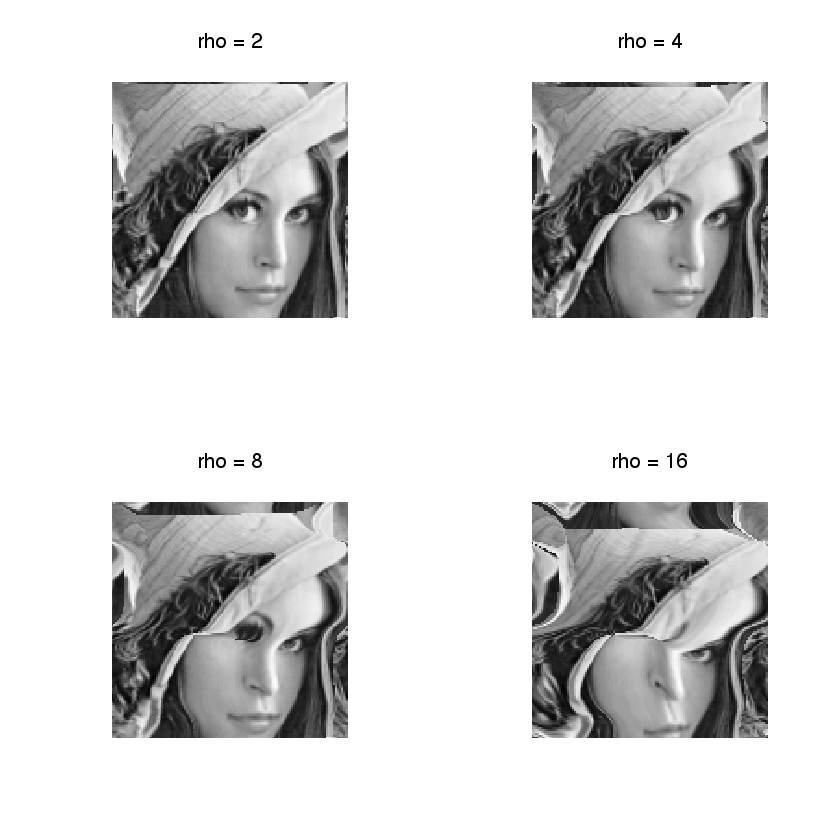In :
## Insert your code here.


Exercise 2

Define an iterative scheme via: $$f^{(\ell+1)} = \Ww_{\rho U}(f^{(\ell)}).$$ Display the result $f^{(\ell)}$, which corresponds approximately to solving an advection equation at time $t=\ell \rho$.

In :
options(repr.plot.width=7, repr.plot.height=7)

source("nt_solutions/graphics_5_fluids/exo2.R")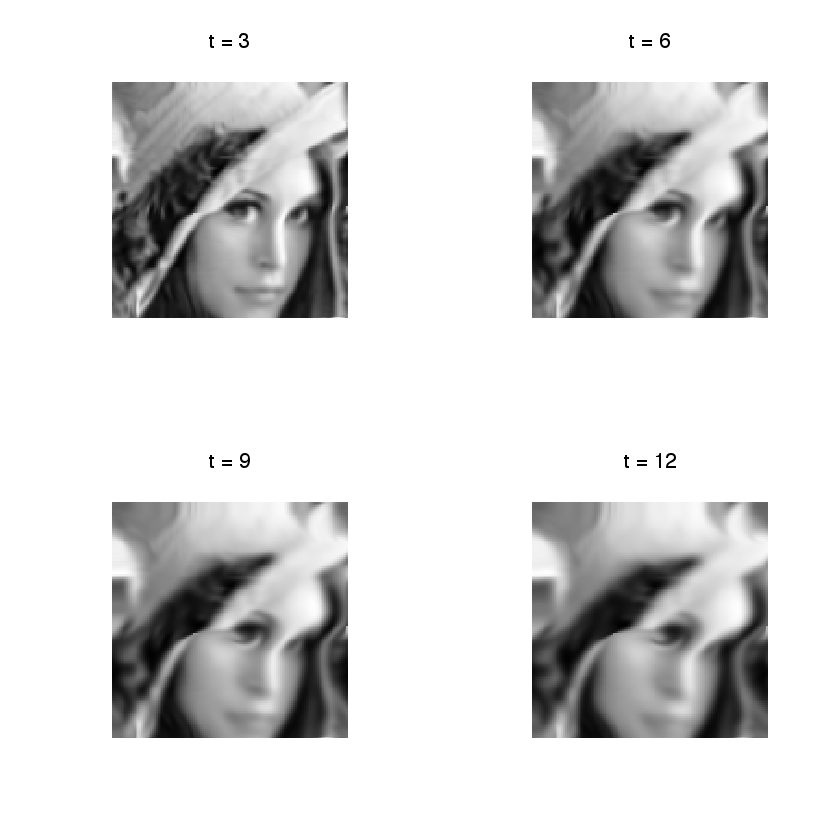In :
## Insert your code here.


## Fluid Dynamics¶

Fluid dynamics solves the incompressible Navier-Stokes equations to evolve in time the vector field.

We discribe here a simple algorithm introduced in:

J. Stam, Stable Fluids, SIGGRAPH'99, 1999, p. 121-128.

It proposes a semi-implicit scheme for the resolution of the Navier Stockes equations for the movement of incompressible fluids $$\pd{V}{t} = \text{Proj}_{\Ii}\pa{ -(V \cdot \nabla) V + \nu \Delta V + W }.$$ Here $\nu \geq 0$ is the viscosity of the fluid, $W$ is a source term, $\Delta$ is the Laplacian, and $-(V \cdot \nabla) V$ is the non-linear self-advection, where we have used the short-hand notation $V \cdot \nabla$ for the derivative operator along a flow $V$: $$(V \cdot \nabla)U = ( V_1 \pd{U_1}{x_1} + V_2 \pd{U_1}{x_2}, V_1 \pd{U_2}{x_1} + V_2 \pd{U_2}{x_2} ).$$

In order to visualize the flow, we also advect and diffuse along the flow a density $g$ of particules, which is a scalar field. Once $V$ has been computed, it follows a linear PDE $$\pd{g}{t} = -(V \cdot \nabla) g + \mu \Delta g + h$$ with some initial condition at time $t=0$, where $h$ is a source for the density.

In practice, we solve this PDE in parallel to the PDE for $V$.

In the following, we use $W=0$ and $h=0$ (no sources).

Set the viscosity $\nu$ for the velocity field.

In :
nu <- 1/10


We use a larger viscosity $\mu$ for the evolution of the density of particules.

In :
mu <- 2*nu


Extend the warping operator $\Ww_U$ to work with vector fields as input. This will apply $\Ww_U$ on each channel of the vector field (X and Y coordinates).

In :
Wt <- function(V, U){ x <- W(V[,,1],U)
y <- W(V[,,2],U)
out <- array(0, dim(V))
out[,,1] <- x ; out[,,2] <- y
return(out)}


We discretize the PDE's using some time step $\tau$.

In :
tau <- .5


The algorithm computes $V^{(\ell)}$ at iteration $\ell$ which is an approximation of the PDE solution at time $\ell \tau$. It is computed itertatively as $$\tilde V^{(\ell)} = \Ww_{\tau V^{(\ell)}}( V^{(\ell)} ) \qandq V^{(\ell+1)} = \text{Proj}_{\Ii}\pa{ \tilde V^{(\ell)} + \tau\nu\Delta \tilde V^{(\ell)} + \tau W }$$

It computes in parallel the evolution of the density as $$\tilde g^{(\ell)} = \Ww_{\tau V^{(\ell)}}( g^{(\ell)} ) \qandq g^{(\ell+1)} = \tilde g^{(\ell)} + \tau\nu\Delta \tilde g^{(\ell)} + \tau h$$

Set the initial field $V=V^{(0)}$ at time $t=0$.

In :
V <- normalize(ProjI(V))


Set the initial density $g=g^{(0)}$ at time $t=0$.

In :
g <- f


The first step is to advect the vector field $V$ and $g$ along the flow $V$ itself. This corresponds to an implict discretization of the term $-(V \cdot \nabla) V$.

In :
g <- W(g, tau*U)
V <- Wt(V, tau*U)


We implement the Laplacian using finite difference.

In :
s1 <- c(2:n,1)
s2 <- c(n,1:(n-1))

Delta <- function(g){
if (length(dim(g))==2) { 1/4.*(g[s1,] + g[s2,] + g[,s1] + g[,s2]) - g }
else if (length(dim(g))==3) { 1/4.*(g[s1,,] + g[s2,,] + g[,s1,] + g[,s2,]) - g } }


The second step is to diffuse the vector field and the density.

In :
V <- V + tau*nu*Delta(V)
g <- g + tau*mu*Delta(g)


The last step is to ensure incompressibility of $V$ by projecting on $\Ii$.

In :
V <- ProjI(V)


Exercise 3

Compute the fluid dynamic by iterating these steps.

In :
options(repr.plot.width=7, repr.plot.height=7)

source("nt_solutions/graphics_5_fluids/exo3.R")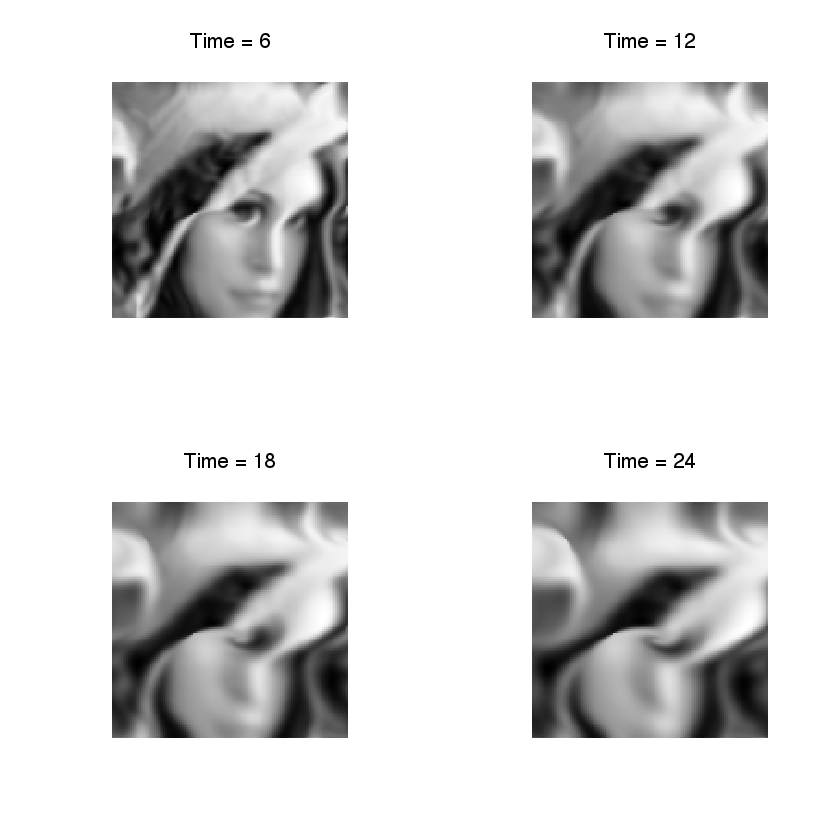In :
## Insert your code here.